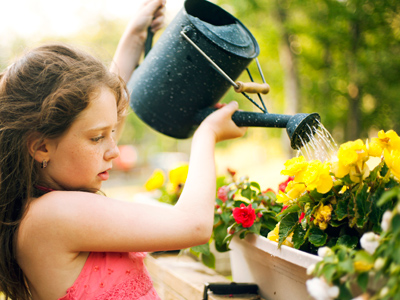If you were investigating the effect different amounts of water had on plant growth, you would need to measure both plant height and amount of water.

# Measurement

This Science quiz is called 'Measurement' and it has been written by teachers to help you if you are studying the subject at elementary school. Playing educational quizzes is an enjoyable way to learn if you are in the 3rd, 4th or 5th grade - aged 8 to 11.

It costs only \$12.50 per month to play this quiz and over 3,500 others that help you with your school work. You can subscribe on the page at Join Us

Measurement usually involves recording the size or length of something, but almost anything can be measured - electrical current, temperature or force for example. Each of these have their own units - electrical current in amps, temperature in degrees Celsius and force in newtons. Accurate measurement is an essential part of undertaking scientific investigations. You will have used many different types of measuring equipment in your lessons. Measuring beakers, meter wheels and stopwatches - these are all pieces of equipment used to measure things. How many more can you remember? And what is each used to measure?

1.
What measuring equipment would you need to measure the height of an object and the amount of a liquid?
A measuring jug and a ruler
A stopwatch and a measuring jug
A ruler and a thermometer
A forcemeter and a ruler
Measuring jugs measure amounts of liquids and rulers measure length, width or height
2.
Which units of measurement would you use for the height of an object and the amount of a liquid?
Amps and newtons
Newtons and kilograms
Grams and degrees Celsius
Millimeters and milliliters
Millimeters can measure height and milliliters can measure amounts of liquid
3.
Which unit is a measurement of volume?
Degrees Celsius
Litres
Metres
Amperes
Degrees Celsius measure temperature, meters measure distance and amperes measure electric current
4.
To investigate the strength of a magnet, you could see how many paper clips could be suspended from the magnet. What would your units be in this investigation?
Metres
Number of paper clips
Litres
Newtons
Force is measured in newtons, using a forcemetre (or Newtonmeter). In this case, however, the number of paper clips would be the unit of measurement
5.
If you were investigating the effect different amounts of water had on plant growth, what would you need to measure?
The plant height only
The amount of water only
Both plant height and amount of water
Neither plant height nor amount of water
As we are looking at the effect different amounts of water have on the height of plants, we need to measure both
6.
Which unit of measurement would you use for temperature?
Degrees Celsius
Litres
Kilograms
Amperes
Temperature is measured in degrees Celsius or sometimes in degrees Fahrenheit or Kelvin
7.
If you were investigating how hand length is related to height, what measuring equipment would you need?
Weighing scales
A measuring jug and a tape measure
A meter rule and a tape measure
A thermometer
You would need to measure both the length of people's hands and their height
8.
Which unit of measurement would you use for length and height?
Degrees Celsius
Litres
Metres
Kilograms
Meters and centimeters are used for measuring length and height
9.
If you created a catapult and wanted to test how far it could 'throw' different objects, what would you need to measure?
The distance the object is 'thrown' and the height of the catapult
The volume and height of the object
The mass of the objects and the distance each object is 'thrown'
All of the above
Lighter objects would probably be thrown further than heavier ones - why not try this experiment to see if I am right?
10.
If you were investigating different materials for insulating coffee, what measuring equipment would you need to test how cool the coffee was after 10 minutes?
A meter rule
A measuring jug
A voltmeter
A thermometer
You would also need a watch or stopwatch to measure the 10 minutes
Author:  Sheri Smith